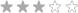Currency - All prices are in AUD

Currency - All prices are in AUDLoading... Please wait...

ARC Welders

• R46,697.00 (inc VAT) R40,606.09 (ex VAT)• R65,075.00 (inc VAT) R56,586.96 (ex VAT)• R192,973.00 (inc VAT) R167,802.61 (ex VAT)• R166,033.00 (inc VAT) R144,376.52 (ex VAT)• R186,648.00 (inc VAT) R162,302.61 (ex VAT)• R36,435.00 (inc VAT) R31,682.61 (ex VAT)• R9,784.00 (inc VAT) R8,507.83 (ex VAT)• R11,337.00 (inc VAT) R9,858.26 (ex VAT)• R22,853.00 (inc VAT) R19,872.17 (ex VAT)• R27,298.00 (inc VAT) R23,737.39 (ex VAT)• R36,285.00 (inc VAT) R31,552.17 (ex VAT)• R7,950.00 (inc VAT) R6,913.04 (ex VAT)• R25,719.00 (inc VAT) R22,364.35 (ex VAT)• R22,753.33 (inc VAT) R19,785.50 (ex VAT)• R4,515.00 (inc VAT) R3,926.09 (ex VAT)• R3,834.00 (inc VAT) R3,333.91 (ex VAT)• R7,553.00 (inc VAT) R6,567.83 (ex VAT)• R4,010.00 (inc VAT) R3,486.96 (ex VAT)• R4,827.00 (inc VAT) R4,197.39 (ex VAT)• R35,590.00 (inc VAT) R30,947.83 (ex VAT)• R27,144.00 (inc VAT) R23,603.48 (ex VAT)• R55,683.00 (inc VAT) R48,420.00 (ex VAT)• R88,998.00 (inc VAT) R77,389.57 (ex VAT)• R17,189.00 (inc VAT) R14,946.96 (ex VAT)• R6,145.00 (inc VAT) R5,343.48 (ex VAT)• R4,422.00 (inc VAT) R3,845.22 (ex VAT)• R5,894.00 (inc VAT) R5,125.22 (ex VAT)• R11,398.86 (inc VAT) R9,912.05 (ex VAT)• R21,374.00 (inc VAT) R18,586.09 (ex VAT)• R3,200.00 (inc VAT) R2,782.61 (ex VAT)